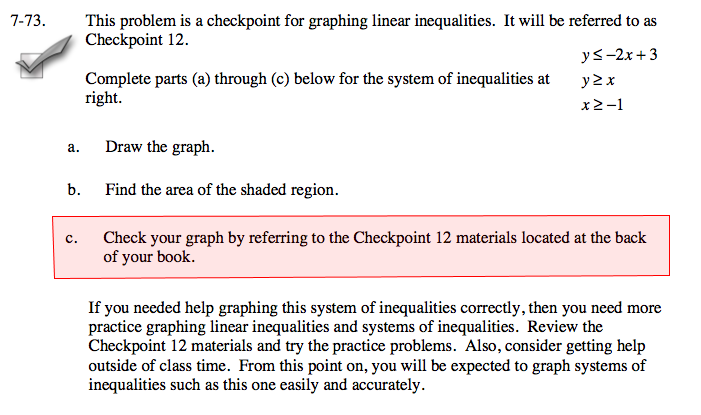### Home > A2C > Chapter 7 > Lesson 7.1.5 > Problem7-73

7-73.
1. This problem is a checkpoint for graphing linear inequalities. It will be referred to as Checkpoint 12. Homework Help ✎Complete parts (a) through (c) below for the system of inequalities below.
y −2x + 3
y x
x ≥ −1

1. Draw the graph.

2. Find the area of the shaded region.

3. Check your graph by referring to the Checkpoint 12 materials located at the back of your book.

If you needed help graphing this system of inequalities correctly, then you need more practice graphing linear inequalities and systems of inequalities. Review the Checkpoint 12 materials and try the practice problems. Also, consider getting help outside of class time. From this point on, you will be expected to graph systems of inequalities such as this one easily and accurately.Answers and extra practice are located in the back of your printed textbook or in the Reference Tab of your eBook.
If you have an eBook for A2C , login and then click the following link: Checkpoint 12 Answers and Practice